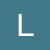Updated by lorileigha on May 22, 2016REPORTlorileigha
Owner
7 items   1 followers   0 votes   56 views

# Systems of Linear Equations

Here are some resources to help you understand how to use and solve systems of linear equations.

1

## Understanding the Basics of Systems of Linear EquationsThis video uses a familiar story about the tortoise and the hare to introduce you to systems of linear equations. After watching this video, you should understand the foundation for how systems of equations can be used to solve problems and what their solutions mean in the context of the situation. The other links will provide you with more details on the methods for solving. This video is only an introduction to the concept. Slow and steady wins the race right? Watch and find out!

2

## Solving Systems of Linear EquationsThis website explains the three types of solutions for systems of linear equations. Hint: they are the same three types that you learned when we solved linear equations before. Can you remember them before going to the link?
There are also three ways to solve systems equations that are all shown, with detailed steps here. Take your time examining the problems. Make sure you can understand each step and could explain the work. Soon you'll be ready to work some of these out on your own.

3

## Using the Substitution MethodThis link focuses on only one of the algebraic methods in detail. Pay close attention to the steps for substituting one equation into the other. If you're confused by just looking at the example, there is a video here as well to help clarify.

5

## Solve Systems by Graphing INTERACTIVE!Move the points on the two lines to graph each equation. Be careful! The equations are in two different forms. If it's in slope-intercept form, first drag a point to the y-intercept and then use the slope (rise/run) to create your next point. But if it's in standard form, solve for the intercepts and plot each point on the correct axis. If you're confused, guess what! There's a video on the right side of the page you can watch. After you graph the lines, find the point of intersection and enter your x and y values to check your answer. See how many you can get right in a row!

6

## Systems of Linear Equations Word ProblemsEach of these problems can be solved by writing and solving a system of linear equations. Start by defining your variables. Then look for your totals. After that, the equations should flow easily from there. Check you answers after you solve and you'll know how you're doing right away.

4

## Using the Elimination Method to Solve Systems of Linear EquationsThis lesson gives several step-by-step examples with elimination. They start out easy by get more challenging as you go so don't skip any examples. Check your understanding by trying to solve the problem on your own before looking at the work, then compare your answers to see how you're doing.

7

## Help with Word ProblemsAlways refer back to these steps if you need help when solving word problems.